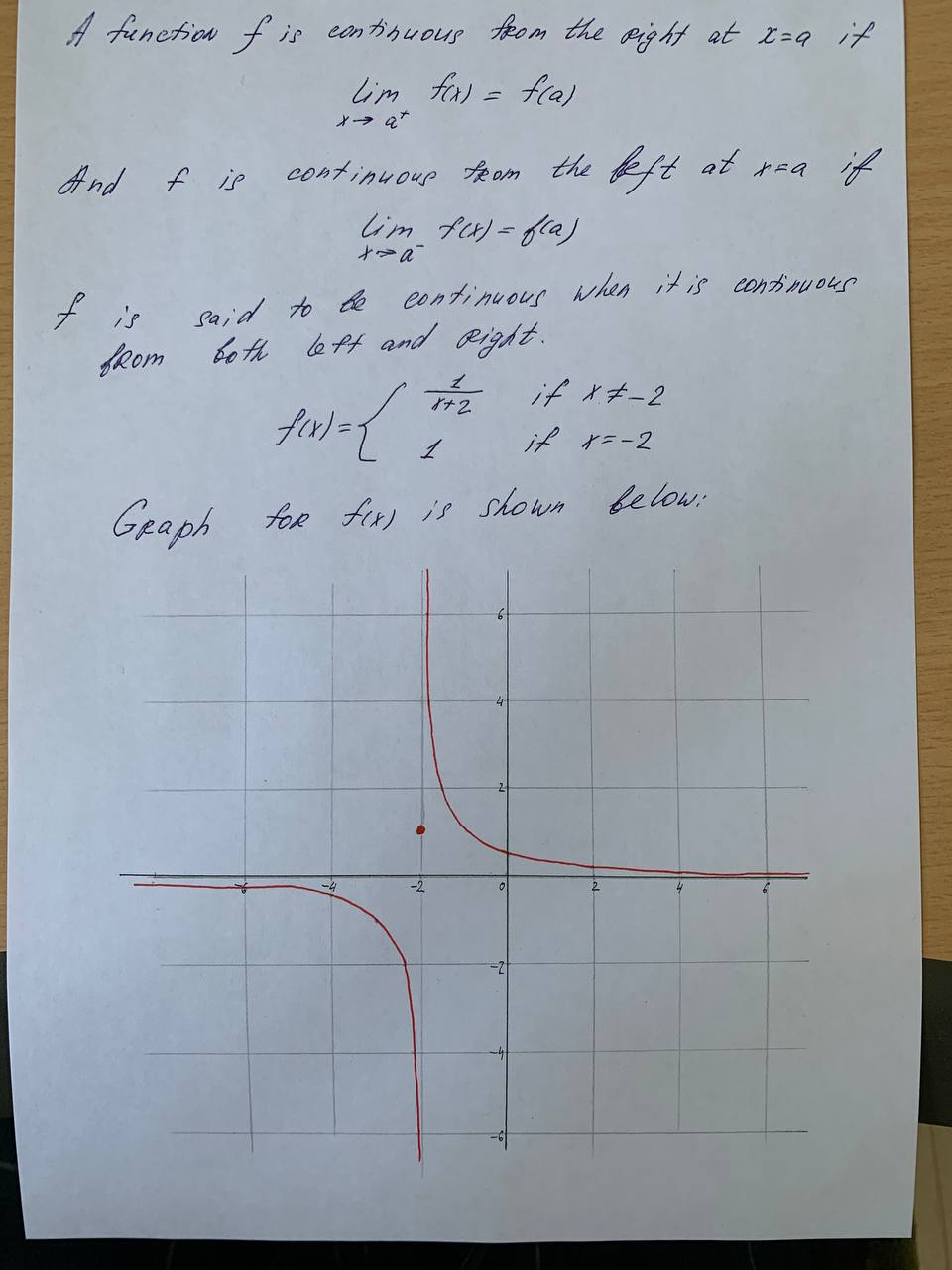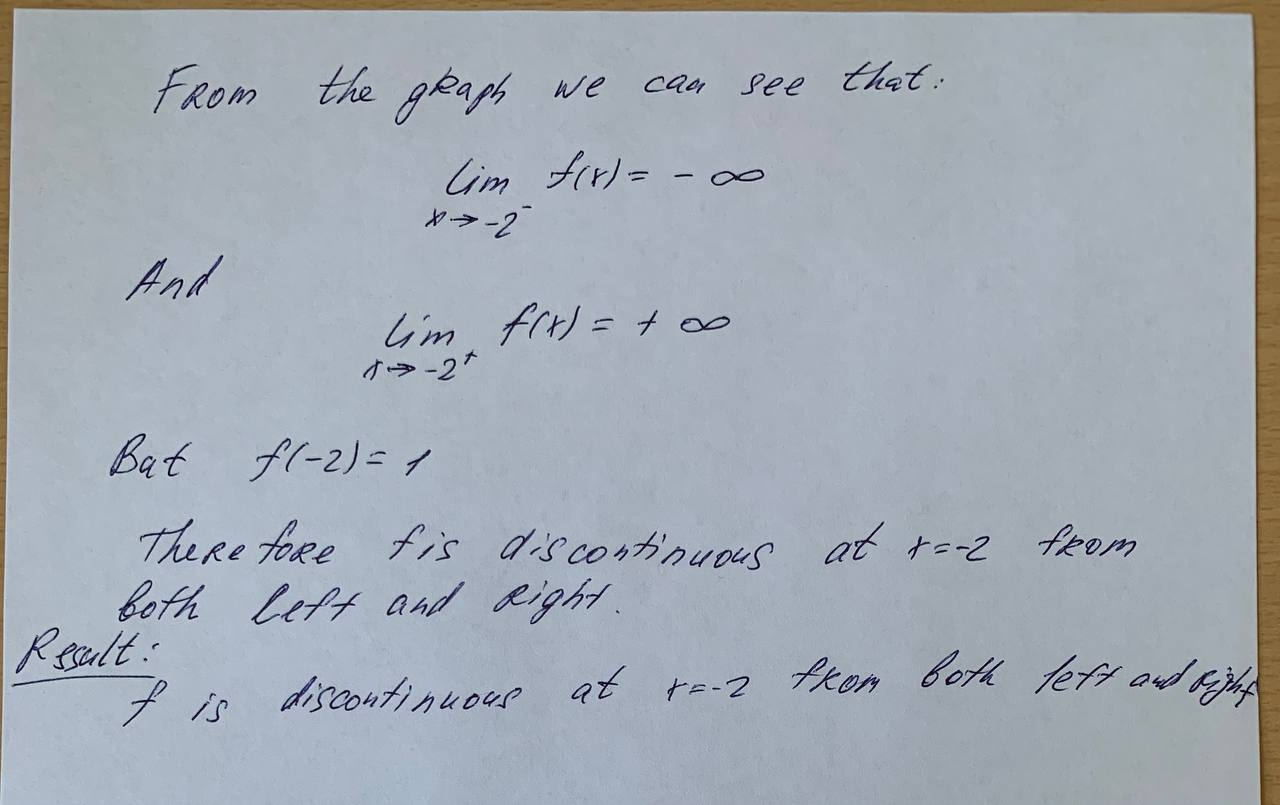Explain why the function is discontinuous at the given number a. Sketch the graph of the function.f(x) = \left\{\frac{1}{x+2}\right\} if x \neq -2 a= -21 if x = -2aflacatn 2021-05-17 Answered

Explain why the function is discontinuous at the given number a. Sketch the graph of the function. $$f(x) = \left\{\frac{1}{x+2}\right\}$$ if $$x \neq -2$$ $$a= -2$$
1 if $$x = -2$$

Want to know more about Laplace transform?

• Questions are typically answered in as fast as 30 minutes

Solve your problem for the price of one coffee

• Math expert for every subject
• Pay only if we can solve itDemi-Leigh BarreraNot exactly what you’re looking for?content_user

A function f is continuous from the right at x=a if

$$\lim_{x\to a^+}f(x)=f(a)$$

And f is continuous from the belt at x=a if

$$\lim_{x\to a^-}f(x)=f(a)$$

f is said to be continuous when it is continuous from both left and right

$$f(x)=\begin{cases}\frac{1}{x+2}&if\ x\ne-2\\1 &if\ x=-2\end{cases}$$

So we can see that:

$$\lim_{x\to-2^-}f(x)=-\infty$$

And

$$\lim_{x\to-2^-}f(x)=+\infty$$

Bat  $$f(-2)=1$$

Therefore fis discontinuous at x=-2 from both left and right

Result: f is discontinuous at x=-2 from both left and right